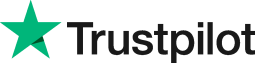How to use the growth formula in Excel
• Home
• Courses
• Promotions
• Schedule
• Formats
• Our Clients

Forum home » Topics » How to use the growth formula in Excel

# How to use the growth formula in Excel

Why use the Growth formula in Excel?
The Growth function can be used to estimate the predicted growth of a business based on past performance.

The calculation is based on a formula that estimates exponential growth. If the past performance suggests that the growth is in a straight line rather than a curve (exponential) then the Excel Trend function can be used instead.

Both the Growth and Trend functions are called Array formulas or CSE formulas. Because of this they need to be entered in a particular way: by pressing the key combination Control + Shift + Enter after typing in the formula.

Here is an example of how the Growth formula can be used:
A B
2006 120
2007 145
2008 175
2009 205
2010 250
2011 300
2012
2013

Suppose these are the number of customers and you want to predict how numbers are growing for 2012 and 2013.

1. Start by highlighting B7:B8.
2. Type =Growth(
3. Highlight B1:B6 (the known y values) then press ,
4. Highlight A1:A6 (the known x values) then press ,
5. Highlight A7:A8 (new x values)
6. Press Ctrl + Shift + Enter

The predicted values are 359 and 431 (rounded to whole numbers)

A way to see the Growth function visually is to create a scatter chart of the data without displaying a line. Then right click a data point and choose Add Trendline. The exponential option uses the Growth function while the Linear option uses the Trend function.

See links below for related used of the Growth formula.

# Related forum posts:

## percentage growth formula

Do you know what formula you type to work out the percentage growth of a series of numbers over a time period? i.e. how much each consecutive figure has increased/decreased by?

# Related articles

## Advanced Excel and PivotTable Reports

 Welcome. Please choose your application (eg. Excel) and then post your question. Our Microsoft Qualified trainers will then respond within 24 hours (working days). Frequently Asked Questions What does 'Resolved' mean? Any suggestions, questions or comments? Please post in the Improve the forum thread.TrustScore 4.8 / 5       1238 reviews